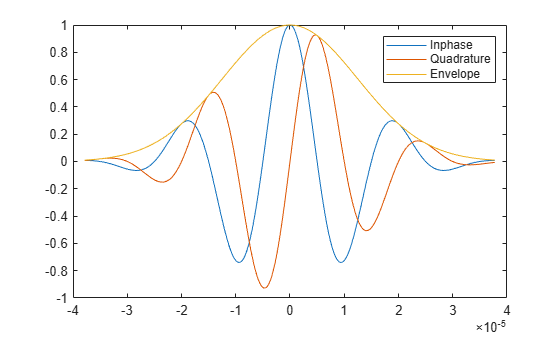Main Content

# gauspuls

Gaussian-modulated sinusoidal RF pulse

## Syntax

``yi = gauspuls(t,fc,bw)``
``yi = gauspuls(t,fc,bw,bwr)``
``[yi,yq] = gauspuls(___)``
``[yi,yq,ye] = gauspuls(___)``
``tc = gauspuls('cutoff',fc,bw,bwr,tpe)``

## Description

````yi = gauspuls(t,fc,bw)` returns a unit-amplitude Gaussian-modulated sinusoidal RF pulse at the times indicated in array `t`, with a center frequency `fc` in hertz and a fractional bandwidth `bw` ```
````yi = gauspuls(t,fc,bw,bwr)` returns a unit-amplitude inphase Gaussian RF pulse with a fractional bandwidth of `bw` as measured at a level of `bwr` dB with respect to the normalized signal peak.```
````[yi,yq] = gauspuls(___)` also returns the quadrature pulse. This syntax can include any combination of input arguments from previous syntaxes.```
````[yi,yq,ye] = gauspuls(___)` returns the RF signal envelope. ```

example

````tc = gauspuls('cutoff',fc,bw,bwr,tpe)` returns the cutoff time `tc` at which the trailing pulse envelope falls below `tpe` dB with respect to the peak envelope amplitude.```

## Examples

collapse all

Plot a 50 kHz Gaussian RF pulse with 60% bandwidth, sampled at a rate of 10 MHz. Truncate the pulse where the envelope falls 40 dB below the peak. Also plot the quadrature pulse and the RF signal envelope.

```tc = gauspuls('cutoff',50e3,0.6,[],-40); t = -tc : 1e-7 : tc; [yi,yq,ye] = gauspuls(t,50e3,0.6); plot(t,yi,t,yq,t,ye) legend('Inphase','Quadrature','Envelope')```## Input Arguments

collapse all

Vector of time values at which the unit-amplitude Gaussian RF pulse is calculated.

Data Types: `single` | `double`

Center frequency of the Gaussian-modulated sinusoidal pulses, specified as a real positive scalar expressed in Hz.

Fractional bandwidth of the Gaussian-modulated sinusoidal pulses,specified as a real positive scalar.

Fractional bandwidth reference level of the Gaussian-modulated sinusoidal pulses, specified as a real negative scalar. `bwr` indicates a reference level less than peak (unit) envelope amplitude. The fractional bandwidth is specified in terms of power ratios. This corresponds to the `-3 dB` point expressed in magnitude ratios.

Trailing pulse envelope level, specified as a real negative scalar in dB. The `tpe` indicates a reference level less than peak (unit) envelope amplitude.

## Output Arguments

collapse all

Inphase Gaussian-modulated sinusoidal pulse, returned as a vector of unit amplitude at the times indicated by the time vector `t`.

Quadrature Gaussian-modulated sinusoidal pulse, returned as a vector of unit amplitude at the times indicated by the time vector `t`.

RF signal envelope of unit amplitude at the times indicated by the time vector `t`.

The cutoff time in seconds at which the trailing pulse envelope falls below `tpe` dB with respect to the peak envelope amplitude.

## See Also

Introduced before R2006a

## Support

#### Deep Learning for Signal Processing with MATLAB

Download white paper